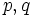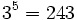# Number of groups of given order

## Definition

Let$n$ be a natural number. The number of groups of order$n$ is defined as the number of isomorphism classes of groups whose order is$n$.

This is a finite number and is bounded by$n^{n^2}$ for obvious reasons. The function is not strictly increasing in$n$ and depends heavily on the nature of the prime factorization of$n$.

## Initial values

The ID of the sequence of these numbers in the Online Encyclopedia of Integer Sequences is A000001

### Numbers up till 100$n$ Number of groups of order$n$ Reason/explanation
1 1
2 1 prime number
3 1 prime number
4 2 square of a prime; see classification of groups of prime-square order
5 1 prime number
6 2 form$pq$ where$p,q$ primes,$q \mid p - 1$
7 1 prime number
8 5 prime cube: classification of groups of prime-cube order, also see groups of order 8
9 2 prime square; see classification of groups of prime-square order
Orders 10 to 36. We omit the prime numbers since there is only one group of each such order.[SHOW MORE] Orders greater than 36. We omit prime numbers, squares of primes, and numbers of the form$pq$ where$p,q$ both primes, since these are covered by standard cases.[SHOW MORE]

### Small powers of small primes

(For general formulas, see the next section).

## Facts

### Basic facts

Value of$n$ What we can say about the number of groups of order$n$ Explanation
1 1 only the trivial group$p$ a prime number 1 only the group of prime order. See equivalence of definitions of group of prime order$p^2$,$p$ prime 2 only the cyclic group of prime-square order and the elementary abelian group of prime-square order$p^3$,$p$ prime 5 see classification of groups of prime-cube order$2^4 = 16$ 14 see classification of groups of order 16, also groups of order 16 for summary information.$p^4$,$p$ odd prime 15 see classification of groups of prime-fourth order for odd prime$2^5 = 32$ 51$3^5 = 243$ 67$p^5$, prime$p \ge 5$$2p + 61 + 2\operatorname{gcd}(p-1,3) + \operatorname{gcd}(p-1,4)$$p^6$, prime$p \ge 5$$3p^2 + 39p + 344 + 24 \operatorname{gcd}(p - 1,3) + 11 \operatorname{gcd}(p-1,4) + 2 \operatorname{gcd}(p-1,5)$
product$p_1p_2 \dots p_n$,$p_i$ distinct primes with no$p_i$ dividing$p_j - 1$ 1 the cyclic group of that order. See classification of cyclicity-forcing numbers
product$pq$,$p,q$ primes with$p$ dividing$q - 1$ 2
product$4p$,$p$ prime,$p > 3$,$p \equiv -1 \pmod 4$ 4
product$4p$,$p$ prime,$p \equiv 1 \pmod 4$ 5

## Properties

### Supermultiplicativity

If$n = ab$ with$a$ and$b$ relatively prime, the number of groups of order$n$ is bounded from below by the product of the number of groups of orders$a$ and$b$ respectively. This is because we can take direct products for every pair of a group of order$a$ and a group of order$b$.